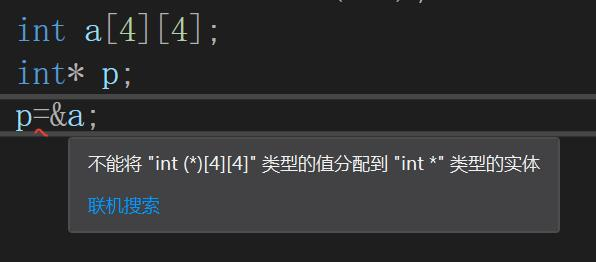Cobb 740 1

查找最长公共子串

#include <iostream>
#include <string>
using namespace std;

// 查找最长公共子串
int main()
{
string str1, str2;
cin >> str1 >> str2;

// 记录最长的公共子串
int max = 0;

for (int i = 0; i < str1.size(); i++)
{
int offset = 0;
for (;;)
{
int j = str2.find(str1[i], offset);
if (j == string::npos)
break;

int size = 0;
for (int m = i, n = j; str1[m] != '\0' && str2[n] != '\0'; m++, n++)
{
if (str1[m] == str2[n])
size++;
else
break;
}

if (size > max)
{
max = size;
}
offset = j + 1;
}

}
cout << max << endl;
}

查找1000以内的完数

int main()
{
vector<int> v;
for (int i = 1; i < 1000; i++)
{
// 判断i是否是完数
int sum = 0;
for (int j = 1; j <= i / 2; j++)
{
if (i % j == 0)
{
sum += j;
v.push_back(j);
// v[k++] = j;
}
}

// 输出打印
if (sum == i)
{
cout << i << "是完数:";
for (int k = 0; k < v.size() - 1; k++)
{
cout << v[k] << ",";
}
// v.back(); 访问容器的最后一个元素
cout << v[v.size() - 1] << endl;
}
v.clear();
}
}

括号匹配(栈)

bool IsSignMatchAll(string signStr)
{
// 括号数量为奇数，则不匹配，直接返回
if (signStr.size() % 2 != 0)
{
return false;
}

stack<char> s;
char cmp;
for (char ch : signStr)
{
if (ch == '{' || ch == '(' || ch == '[')
{
s.push(ch);
}
else
{
switch (ch)
{
case '}':
cmp = '{';
break;
case ')':
cmp = '(';
break;
case ']':
cmp = '[';
break;
}
if (s.empty())
return false;
char top = s.top();
s.pop();
if (top != cmp)
return false;
}
}

return s.empty();
}

笔试面试题目：阿里选班长

方案三：摩尔投票

//不相同的就抵消，相同的计数+1
#include <iostream>
using namespace std;

int solution(int a[], int n) {
int count = 0, value = a;
for(int i = 0; i < n; i++)
{
printf("log, i=%d, count=%d, value=%d\n", i, count, value);
if(count == 0)
{
value = a[i];
}

if(a[i] == value)
{
count++;
}
else
{
count--;
}
}

return value;
}

int main()
{
int a[]={6,2,5,3,4,2,2,2,2,5};
int n = sizeof(a) / sizeof(a);
cout << solution(a, n) << endl;
return 0;
}

log, i=0, count=0, value=6
log, i=1, count=1, value=6
log, i=2, count=0, value=6
log, i=3, count=1, value=5
log, i=4, count=0, value=5
log, i=5, count=1, value=4
log, i=6, count=0, value=4
log, i=7, count=1, value=2
log, i=8, count=2, value=2
log, i=9, count=3, value=2
2

C++11的LAMBDA使用一例：华容道求解

C++11的LAMBDA使用一例：华容道求解

指针数组和数组指针

![2N4ZVL(K[52_VP7V~%O_MC3. int a; 问下面哪些不可以表示 a 的地址？ （ A ）
A. a+sizeof(int)
B. &a+1
C. (int*)&a+1
D. (int*)((char*)&a+sizeof(int))
/*

Type* a;
a + n;   &a ==> (unsigned int)a + 4   // a是个int型，所以这是a的地址

&a ==》 int(*)   数组类型
a  ==》 int*     指针类型

(unsigned int)a + n * sizeof(Type)
A. (unsigned int)a + 4 * 4
B. (unsigned int)(&a) + 1 * 4
C. (int*)&a 转成 a ==> a + 1 ==> a
D. (unsigned int)a + 4 * 1

*/